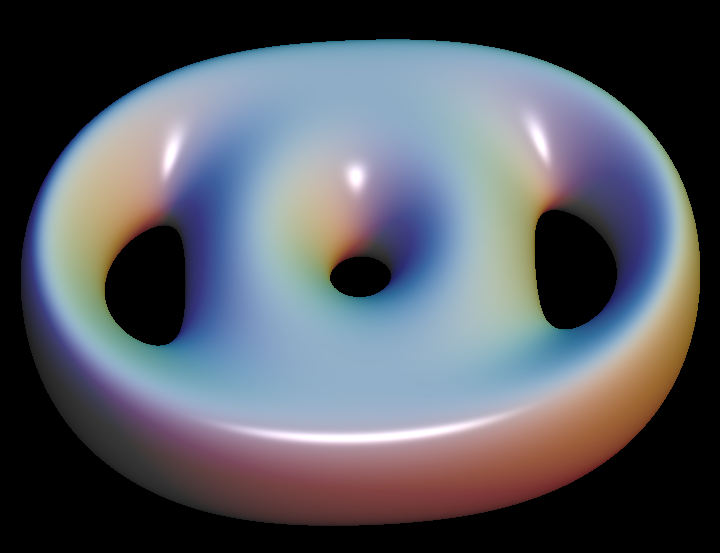# Pretzel Surface6 different levels of the implicit rational function for Pretzel, f(x,y,z) = c for 6 values of c.

The Pretzel surface is an algebraic surface given by

`f(x,y,z) := (((x-1)^2 + y^2 - aa^2) * ((x+1)^2 + y^2 - aa^2))^2 + z^2`